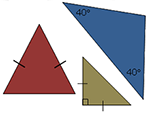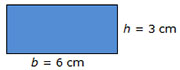Properties of Geometric Figures

My Definition

Key Characteristics

The following are true of properties of geometric figures:

• The term refers to common characteristics of a group or class of figures.

• They can be used to classify geometric figures.

Example

A property of all parallelograms is that the diagonals bisect each other.Use the link below to further study examples of Properties of Geometric Figures.

Properties of Geometric Figures ActivityNon-exampleThe given dimensions are measureable attributes of this particular rectangle.

TEKS: 2(8)(D), 4(6)(B), 4(6)(D), 5(5)(A), 6(8)(A)